NEET  >  Rate of a Chemical Reaction and Factors Affecting Rate

# Rate of a Chemical Reaction and Factors Affecting Rate - Notes | Study Chemistry Class 12 - NEET

 Table of contentsRate of a ReactionWhat is Chemical Kinetics?Factors Affecting the Rate of Reaction1 Crore+ students have signed up on EduRev. Have you?

Rate of a Reaction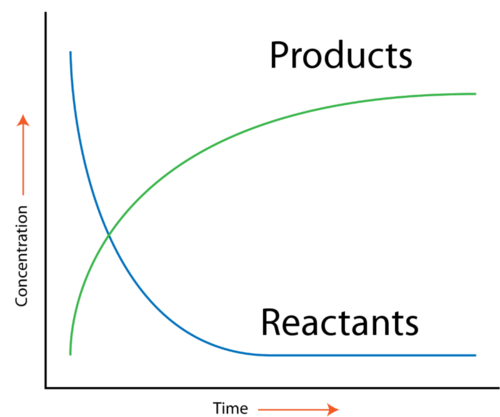• The rate of reaction refers to the speed at which the products are formed from the reactants in a chemical reaction.
• It gives some insight into the time frame under which a reaction can be completed. For example, the reaction rate of the combustion of cellulose in fire is very high and the reaction is completed in less than a second.
• In general, for a reaction : R → P, the behaviour of the concentration of the reactant and product, as the reaction proceeds is shown graphically: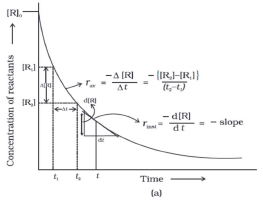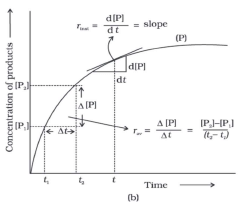From the graph, it is clear that the concentration of the reactant decreases and that of the product increases as the reaction proceeds and the rate of the change of the concentration of the reactant as well as that of the product is also changing.

Rate of a reaction can, now, be defined in two ways:

Average Rate of reaction (rav) given by for the reaction R → P:

rav =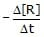=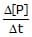where Δ[R] and Δ[P] represents the change in the concentrations of 'R' and 'P' respectively over a time interval Δt

The average rate of the reaction between a time interval (tf - tΔt) can be determined from the above graph by locating the concentration of 'R' of 'P' on this graph at the time instants tf and ti as shown.

If [R]f and [R]i are the concentrations of the reactant 'A' at the time instants tf and ti then :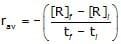Similarly from the plot of 'P' as a function of 't', we have :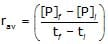Note:

• The above expression for rav is equivalent to the slope of the line joining the points (t[A]f) and (t[R]i) or (t[P]f) and (t[P]i) as shown.

Instantaneous Rate of reaction (rinst.) can be calculated from rav

in the limit Δt → 0 and is represented as :

rinst. =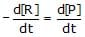Note:

• The above expression for rinst. is equivalent to the slope of the tangent from the plot of the concentration of 'R' or 'P' at any time instant 't'.
•  The rate of the reaction (rinst. or rav) is always calculated as a positive quantity.
• The rate of a reaction at any temperature depends on the concentration of the reactants and sometimes on the concentration of some foreign substances (e.g a catalyst) being used in the reaction as well. The representation of this dependence of the rate of the reaction on the concentrations is known as rate law and this rate law is determined experimentally.

Units of rate of a reaction:

Units of rate are concentration time-1. For example, if concentration is in mol L-1 and time is in seconds then the units will be mol L-1s-1. However, in gaseous reactions, when the concentration of gas is expressed in terms of their partial pressures, then the units of the rate equation will be atm s-1.

Relation between various rates:

In general for a reaction : aA + bB → cC + dD

The rate of reaction can be expressed as follows :

Rate =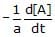=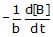=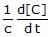=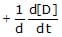= kr[A]m[B]n

What is Chemical Kinetics?

This branch of chemistry which deals with the study of rates of chemical reactions and the mechanism by which they occur. While studying reaction, one deals with:

(a) how fast (or slow) the reactants get converted into products

(b) the steps or paths through which the products are formed (reaction mechanism)

Chemical reaction kinetics deals with the rates of chemical processes. Any chemical process may be broken down into a sequence of one or more single-step processes known either as elementary processes, elementary reactions, or elementary steps.
Elementary reactions usually involve either a single reactive collision between two molecules, which we refer to as a a bimolecular step, or dissociation/isomerization of a single reactant molecule, which we refer to as a unimolecular step.
Very rarely, under conditions of extremely high pressure, a termolecular step may occur, which involves simultaneous collision of three reactant molecules. An important point to recognize is that many reactions that are written as a single reaction equation in actual fact consist of a series of elementary steps. This will become extremely important as we learn more about the theory of chemical reaction rates.

As a general rule, elementary processes involve a transition between two atomic or molecular states separated by a potential barrier. The potential barrier constitutes the activation energy of the process, and determines the rate at which it occurs. When the barrier is low, the thermal energy of the reactants will generally be high enough to surmount the barrier and move over to products, and the reaction will be fast. However, when the barrier is high, only a few reactants will have sufficient energy, and the reaction will be much slower.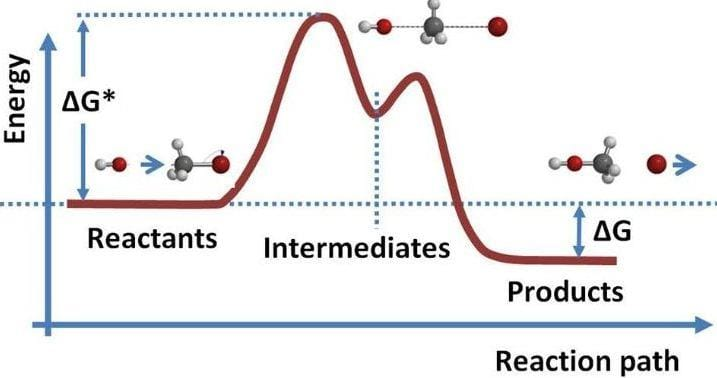Fig: Chemical kinetics

The presence of a potential barrier to reaction is also the source of the temperature dependence of reaction rates. The huge variety of chemical species, types of reaction, and the accompanying potential energy surfaces involved means that the timescale over which chemical reactions occur covers many orders of magnitude, from very slow reactions, such as iron rusting, to extremely fast reactions, such as the electron transfer processes involved in many biological systems or the combustion reactions occurring in flames.
A study into the kinetics of a chemical reaction is usually carried out with one or both of two main goals in mind:

1. Analysis of the sequence of elementary steps giving rise to the overall reaction. i.e. the reaction mechanism.

2. Determination of the absolute rate of the reaction and/or its individual elementary steps.

Factors Affecting the Rate of Reaction

The various factions that can affect the rate of a chemical reaction are listed in this subsection.

Nature of the reaction

• The rate of reaction highly depends on the type and nature of the reaction. As mentioned earlier, few reactions are naturally faster than others while some reactions are very slow.
• The physical state of reactants, number of reactants, complexity of reaction and other factors highly influence the reaction rate as well.
• The rate of reaction is generally slower in liquids when compared to gases and slower in solids when compared to liquids. Size of the reactant also matters a lot. The smaller the size of reactant, the faster the reaction.

Effect of concentration on reaction rate

• According to the collision theory, the rate of reaction increases with the increase in the concentration of the reactants.
• As per the law of mass action, the chemical reaction rate is directly proportional to the concentration of reactants.
• This implies that the chemical reaction rate increases with the increase in concentration and decreases with the decrease in the concentration of reactants.
• Time plays a major role in changing the concentration of reactants and products. Therefore, even time is a vital factor affecting the reaction rate.

Pressure factor

• Pressure increases the concentration of gases which in turn results in the increase of the rate of reaction. The reaction rate increases in the direction of less gaseous molecules and decreases in the reverse direction.
• Thus, it can be understood that pressure and concentration are interlinked and that they both affect the rate of reaction.

How does temperature affect the reaction rate?

• According to collision theory, a chemical reaction that takes place at a higher temperature generates more energy than a reaction at a lower temperature.
• This is because colliding particles will have the required activation energy at high temperature and more successful collisions will take place.
• There are some reactions that are independent of temperature. Reactions without an activation barrier are examples of chemical reactions that are independent of temperature.

Solvent

The rate of reaction also depends on the type of solvent. Properties of solvent and ionic strength highly affect the reaction rate.

Order

The order of reaction manages how the reactant pressure or concentration affects the rate of reaction.

Electromagnetic radiation is a form of energy and its presence at the chemical reaction may increase the rate of reaction as it gives the particles of reactants more energy.

Intensity of Light

Even the intensity of light affects the rate of reaction. Particles absorb more energy with the increase in the intensity of light thereby increasing the rate of reaction.

Presence of Catalyst

• A catalyst can be defined as a substance that increases the rate of the reaction without actually participating in the reaction. The definition itself describes its effect on chemical reactions.
• The presence of a catalyst increases the speed of reaction in both forward and reverse reaction by providing an alternate pathway which has lower activation energy.

Surface Area of the Reactants

The surface area of reactants affects the rate of reaction. If the size of a particle is small, the surface area will be more and this increases the speed of heterogeneous chemical reactions.

The document Rate of a Chemical Reaction and Factors Affecting Rate - Notes | Study Chemistry Class 12 - NEET is a part of the NEET Course Chemistry Class 12.
All you need of NEET at this link: NEET

## Chemistry Class 12

157 videos|385 docs|261 tests

## Chemistry Class 12

157 videos|385 docs|261 tests

### How to Prepare for NEET

Read our guide to prepare for NEET which is created by Toppers & the best Teachers

Track your progress, build streaks, highlight & save important lessons and more!

,

,

,

,

,

,

,

,

,

,

,

,

,

,

,

,

,

,

,

,

,

;